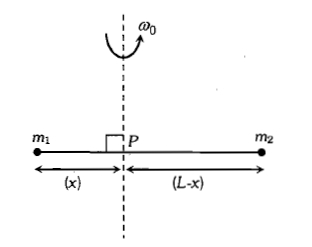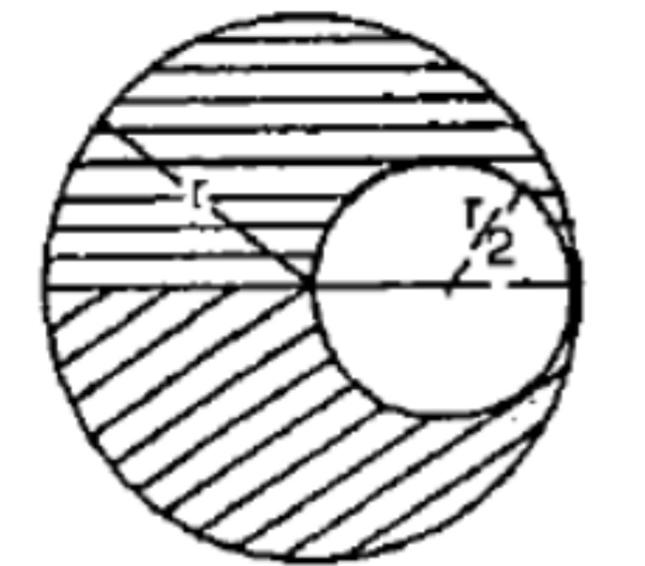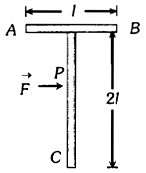If the net external forces acting on the system of particles is zero, then which of the following may vary ?

1. Momentum of the system

2. Velocity of centre of mass

3. Position of centre of mass

4. None of the above

Concept Questions :-

Center of mass
High Yielding Test Series + Question Bank - NEET 2020

Difficulty Level:

A constant torque of 1000 N-m turns a wheel of moment of inertial 200 $\mathrm{kg}-{\mathrm{m}}^{2}$ about an axis through its centre. Its angular velocity after 3 s is

Concept Questions :-

Rotation motion : Introduction
High Yielding Test Series + Question Bank - NEET 2020

Difficulty Level:

Point masses ${\mathrm{m}}_{1}$ and ${\mathrm{m}}_{2}$ are placed at the opposite ends of a rigid of length L and negligible mass. The rod is to be set rotating about an axis perpendicular to it. The position of point P on this rod through which the axis should pass so that the work required to set the rod rotating with angular velocity ${\mathrm{\omega }}_{0}$ is minimum is given byConcept Questions :-

Moment of inertia
High Yielding Test Series + Question Bank - NEET 2020

Difficulty Level:

A circular disc of radius r has a uniform thickness.  A circular hole of diameter equal to the radius of the disc has been cut out as shown.  The centre of gravity of the remaining disc lies on the diameter of the disc at a distance x to the left of the centre of the original disc.  The value of x is(1) r                              (2) $\frac{r}{2}$

(3) $\frac{r}{4}$                           (4) $\frac{r}{6}$

High Yielding Test Series + Question Bank - NEET 2020

Difficulty Level:

A 'T' shaped object with dimensions shown in the figure, is lying on a smooth floor. A force '$\stackrel{\to }{\mathrm{F}}$' is applied at the point P parallel to AB, such that the object has only the translational motion without rotation. Find the location of P with respect to C1.  $\frac{4}{3}l$

2.  I

3.  $\frac{2}{3}l$

4.  $\frac{3}{2}l$

Concept Questions :-

Center of mass
High Yielding Test Series + Question Bank - NEET 2020

Difficulty Level:

A wheel is rotating about an axis through its centre at 720 r.p.m. It is acted on by a constant torque opposing its motion for 8 seconds to bring it to rest finally. The value of torque is Nm is (given l = $\frac{24}{\mathrm{\pi }}$ kg ${\mathrm{m}}^{2}$)

1. 48

2. 72

3. 96

4. 120

Concept Questions :-

Torque
High Yielding Test Series + Question Bank - NEET 2020

Difficulty Level:

Concept Questions :-

Rolling motion
High Yielding Test Series + Question Bank - NEET 2020

Difficulty Level:

Assertion : The speed of whirlwind in a tornado is alarmingly high.

Reason    : If no external torque acts on a body, its angular velocity remains conserved.

(1) If both assertion and reason are true and reason is the correct explanation of assertion.

(2) If both assertion and reason are true but reason is not the correct explanation of assertion.

(3) If assertion is true but reason is false.

(4) If both assertion and reason are false.

Concept Questions :-

Angular momentum
High Yielding Test Series + Question Bank - NEET 2020

Difficulty Level:

A circular disc A of radius r is made from an iron plate of thickness t and another circular disc B of radius 2r and thickness $\frac{\mathrm{t}}{4}$. The relation between moments of inertia ${\mathrm{I}}_{\mathrm{A}}$ and ${\mathrm{I}}_{\mathrm{B}}$ is

1.

2.

3.

4.  Depends on the actual values of t and r

Concept Questions :-

Moment of inertia
High Yielding Test Series + Question Bank - NEET 2020

Difficulty Level:

A stationary bomb explodes into two parts of masses 3kg and 1kg. The total KE of the two parts after explosion is 2400 J. The KE of smaller part is

1. 600 J

2. 1800 J

3. 1200 J

4. 2160 J

Concept Questions :-

Linear momentum